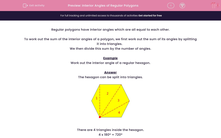# Interior Angles of Regular Polygons

In this worksheet, students calculate the interior angle of regular polygons.This content is premium and exclusive to EdPlace subscribers.Key stage:  KS 2

Curriculum topic:   Maths and Numerical Reasoning

Curriculum subtopic:   2D Shapes: Triangles, Quadrilaterals and Polygons

Difficulty level:#### Worksheet Overview

Regular polygons have interior angles which are all equal to each other.

To work out the sum of the interior angles of a polygon, we first work out the sum of its angles by splitting it into triangles.

We then divide this sum by the number of angles.

Example

Work out the interior angle of a regular hexagon.

The hexagon can be split into triangles.There are 4 triangles inside the hexagon.

4 x 180º = 720º

The sum of the interior angles of a hexagon is 720º.

There are 6 equal angles inside the hexagon.

Each interior angle is 720º ÷ 6 = 120º

### What is EdPlace?

We're your National Curriculum aligned online education content provider helping each child succeed in English, maths and science from year 1 to GCSE. With an EdPlace account you’ll be able to track and measure progress, helping each child achieve their best. We build confidence and attainment by personalising each child’s learning at a level that suits them.

Get started